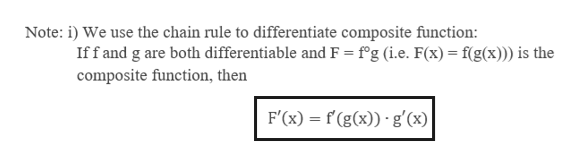# Logarithmic Differentiation of (ln x)^x^2

Question

Logarithmic Differentiation of (ln x)^x^2

check_circleExpert Solution
Step 1

Given expression

Step 2

In implicit differentiation, we differentiate each side of an equation by treating one of the variables as a function of the other. We treat y as an implicit function of x.help_outlineImage TranscriptioncloseNote: i) We use the chain rule to differentiate composite function: Iff and g are both differentiable and F = f'g (i.e. F(x)= f(g(x))) is the composite function, then F'(x) f(g(x)) g'(x) fullscreen
Step 3

To begin with:...

### Want to see the full answer?

See Solution

#### Want to see this answer and more?

Solutions are written by subject experts who are available 24/7. Questions are typically answered within 1 hour*

See Solution
*Response times may vary by subject and question
Tagged in

### Calculus